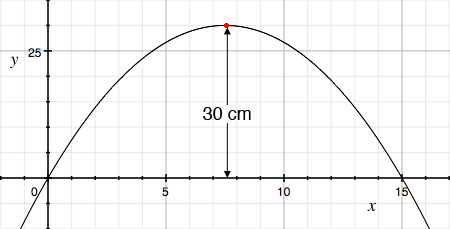SEARCH HOMEMath Central Quandaries & QueriesQuestion from Hannah, a student: A flea jumps from the ground to a height of 30 cm and travels 15 cm horizontally from where it started. Suppose the origin is located at the point from which the flea jumped. Determine a quadratic function in vertex form to model the height of the flea compared to the horizontal distance travelledHi Hannah,

Here is my diagram of the quadratic function.From the diagram the quadratic has roots at $x = 0$ and $x = 15.$ What is the equation of a quadratic with roots of $x = 0$ and $x = 15?$ What is another quadratic with these roots? It might help to look here or here. What is the equation of a quadratic with roots of $x = 0$ centimeters and $x = 15$ centimeters and maximum value of $f(x) = 30$ centimeters?

PennyMath Central is supported by the University of Regina and the Imperial Oil Foundation.